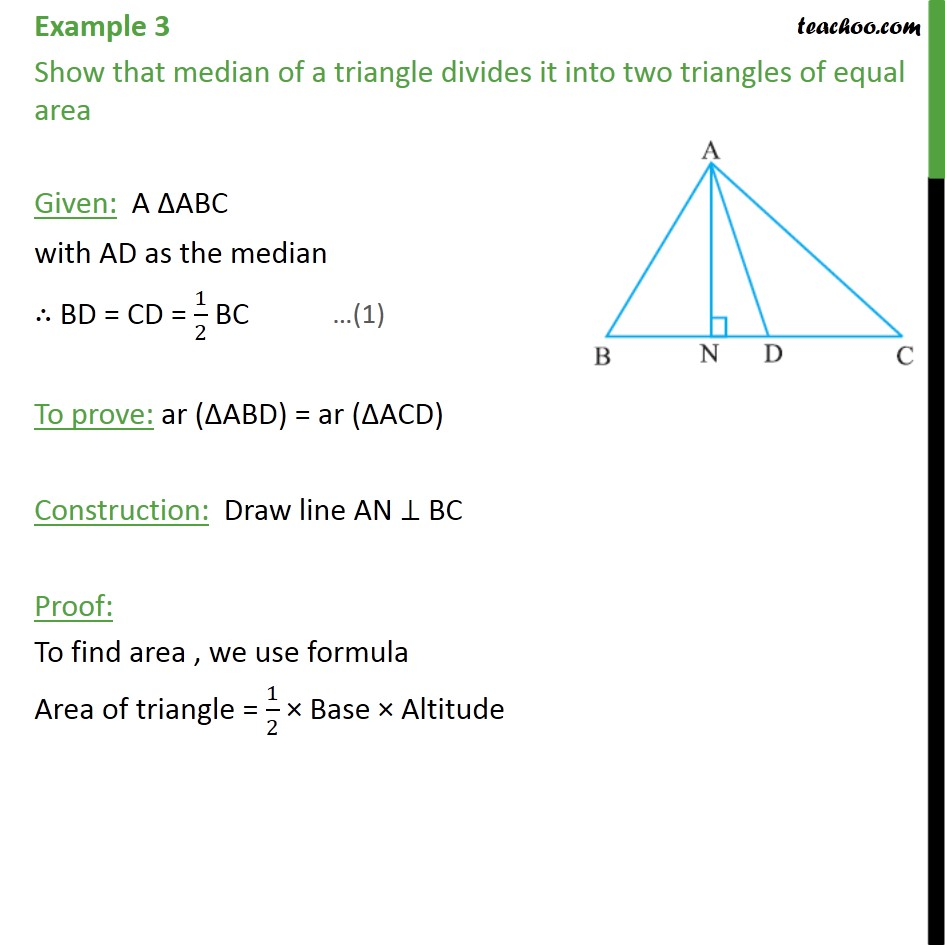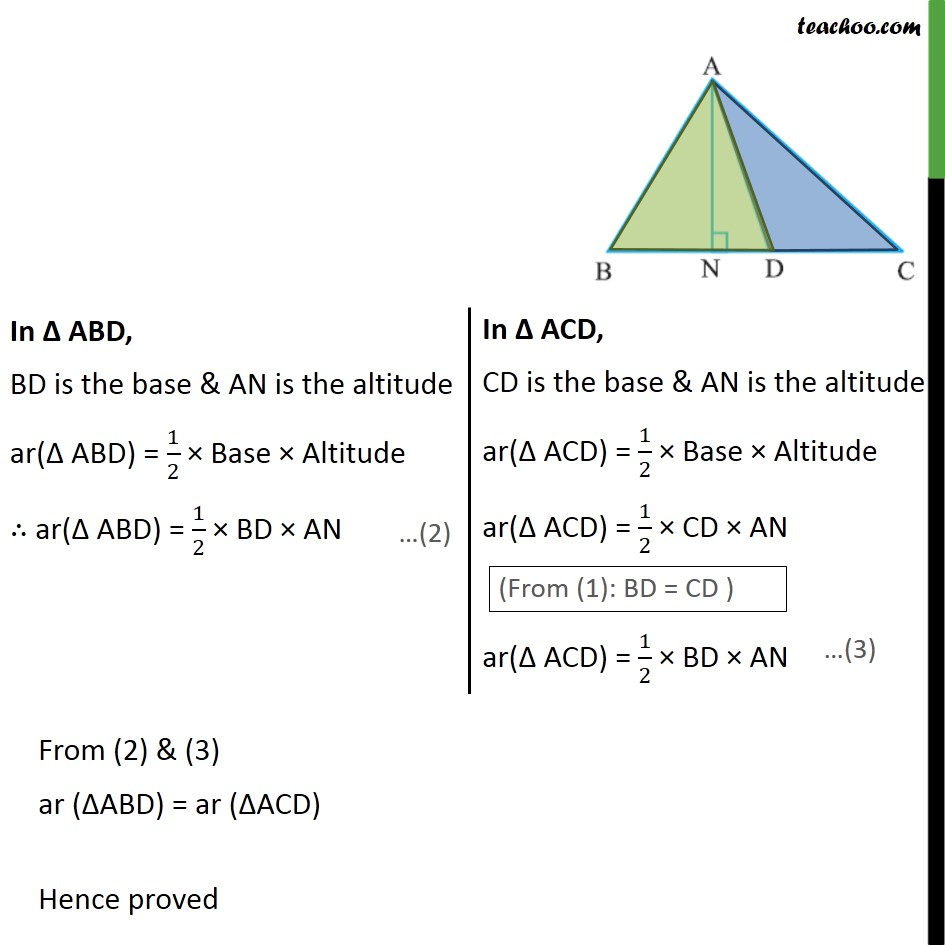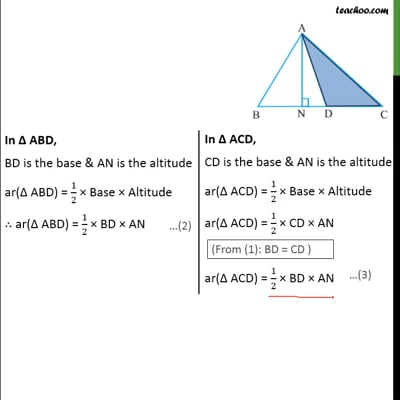Examples

Chapter 9 Class 9 - Areas of Parallelograms and Triangles (Deleted)
Serial order wiseThis video is only available for Teachoo black users

### Transcript

Example 3 Show that median of a triangle divides it into two triangles of equal area Given: A ABC with AD as the median BD = CD = 1/2 BC To prove: ar ( ABD) = ar ( ACD) Construction: Draw line AN BC Proof: To find area , we use formula Area of triangle = 1/2 Base Altitude From (2) & (3) ar ( ABD) = ar ( ACD) Hence proved